# Details for `confinement-measures-combined-incl-overland-spotify.ipynb`

#### Description

How did my behaviour change during the 2-month long COVID-19 lockdown in France? We'll dive into some of the numbers and compare it to before/after

#### Tags & Data Sources

lockdown covid19 behavior change Oura Connect RescueTime connection Spotify integration Overland connection

# Lockdown effects¶

If you want to run this notebook and run into problems or have questions: Reach out to Bastian on Twitter or Slack

France was on COVID-19 lockdown from mid-March until mid-May. How did my behaviour change over those two months? Let's have a look at data on

• productivity
• physical activity
• sleep
• music listening habits
• movement behaviour

This notebook makes use of data from a variety of sources. If you want to run this analysis for your data you need the following data sources connected to your Open Humans account (or selectively run it):

## Plotting the effects¶

There will be some inherent noise in day-to-day measures as behaviour changes depending on the day of the week (just think of weekdays vs weekends) To remove a good bit of this variance I'm taking only the weekly mean values (in case of sleep/activity) or the sum of all values within a week (in case of productivity).

```/opt/conda/lib/python3.7/site-packages/rpy2/robjects/pandas2ri.py:14: FutureWarning: pandas.core.index is deprecated and will be removed in a future version.  The public classes are available in the top-level namespace.
from pandas.core.index import Index as PandasIndex
```
```WARNING:rpy2.rinterface_lib.callbacks:R[write to console]:
Attaching package: ‘lubridate’

WARNING:rpy2.rinterface_lib.callbacks:R[write to console]: The following objects are masked from ‘package:base’:

date, intersect, setdiff, union

WARNING:rpy2.rinterface_lib.callbacks:R[write to console]:
********************************************************

WARNING:rpy2.rinterface_lib.callbacks:R[write to console]: Note: As of version 1.0.0, cowplot does not change the

WARNING:rpy2.rinterface_lib.callbacks:R[write to console]:   default ggplot2 theme anymore. To recover the previous

WARNING:rpy2.rinterface_lib.callbacks:R[write to console]:   behavior, execute:
theme_set(theme_cowplot())

WARNING:rpy2.rinterface_lib.callbacks:R[write to console]: ********************************************************

WARNING:rpy2.rinterface_lib.callbacks:R[write to console]:
Attaching package: ‘cowplot’

WARNING:rpy2.rinterface_lib.callbacks:R[write to console]: The following object is masked from ‘package:lubridate’:

stamp

```

Warning: These widgets currently don't work in the Voila display as they use a hacky Javascript solution to re-run the notebook. If you want to edit those values you unfortunately have to do so from the regular Jupyter Notebook interface

## Effect on Productivity (as measured by RescueTime)¶

We start off by plotting the RescueTime data. RescueTime gives us some details on which categories the time is spent in, but we'll define some extra categories, which are meetings (via Zoom, Google Meet, Jitsi etc. as well as in-person meetings) and messages, which would be Slack, Mail, Rocket.Chat etc.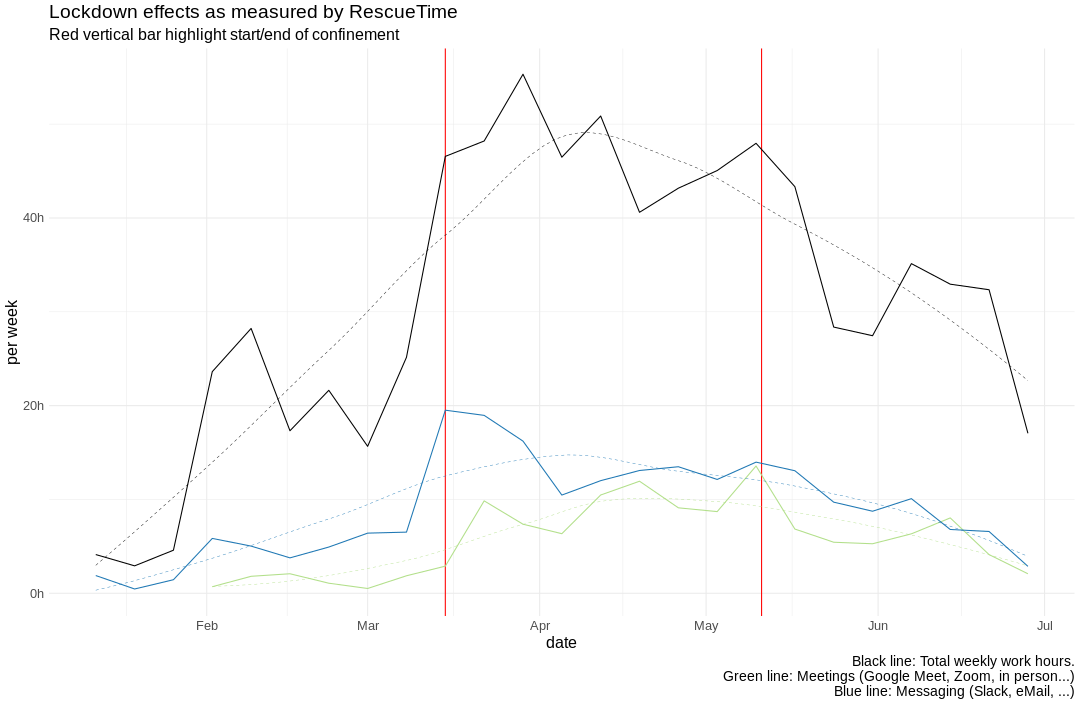The plot divides the time in before and after the lockdown, with everything in between the two red bars being the lockdown period. The dashed lines are smoothed, while the continuous lines give the individual weekly data points. There are some clear differences to be seen.

# Effect on Activity & Sleep (Oura)¶

Let's also check out the sleep and physical activity for the lockdown, here taken from the Oura ring.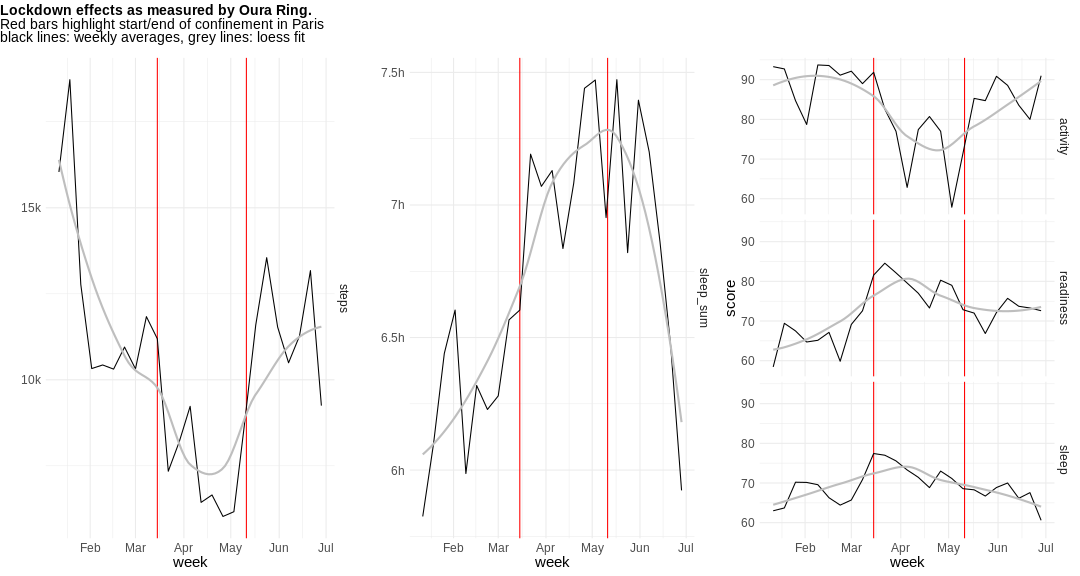We plot both the raw data for the average nightly sleep time and the average number of steps for a week and plot those, as well as some processed "scores" that Oura assigns, which range between 0-100, with 100 being "the best". Looks like the post-lockdown life is returning pretty much to normal. For good (in terms of physical activity) but also for bad (in terms of sleep)

# Did the confinement change how much I listen to music?¶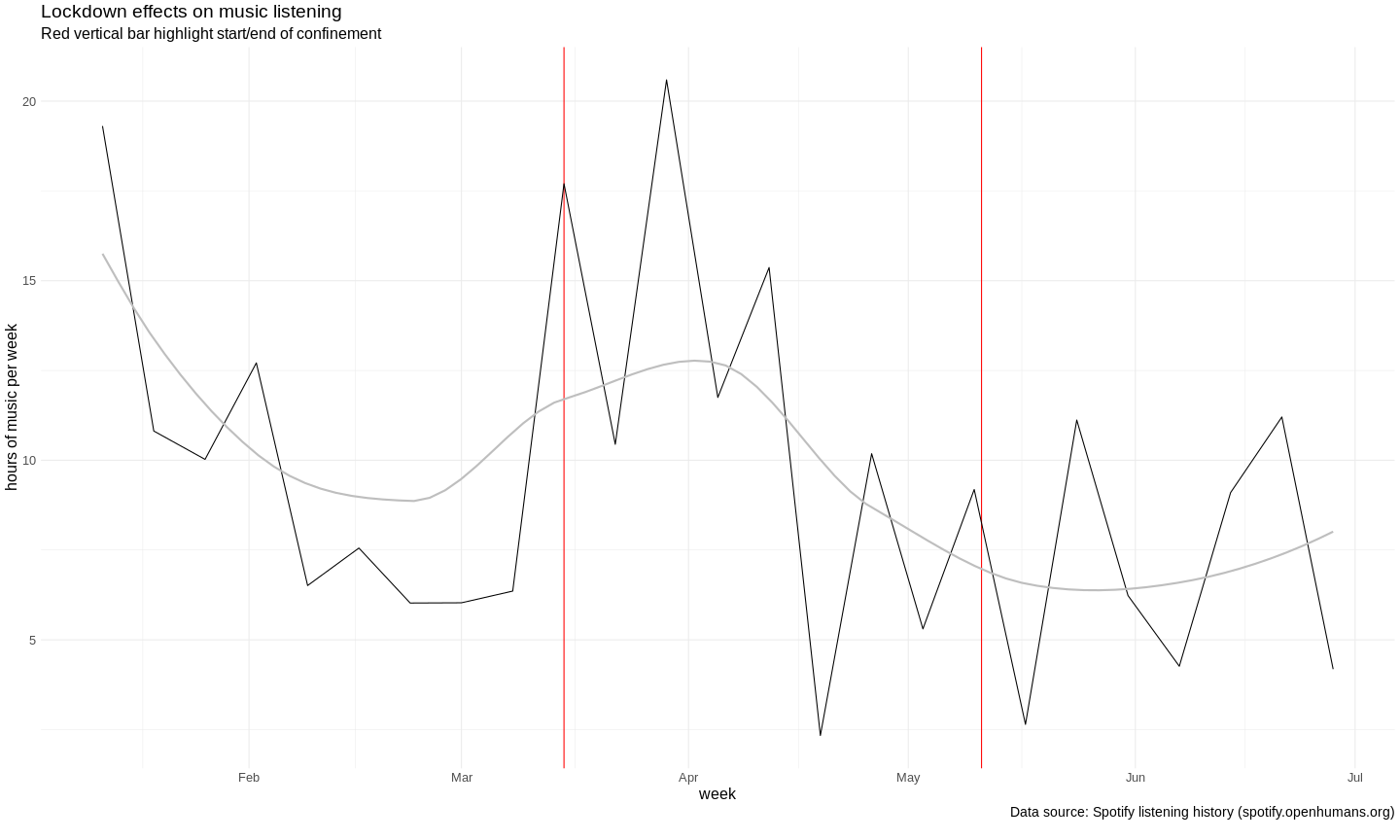# Effects on Movement¶

Thanks to Overland I also have a full history of how much I moved, regardless of the mode of transportation (walking, biking, public transport, …). How does the lockdown effect appear there?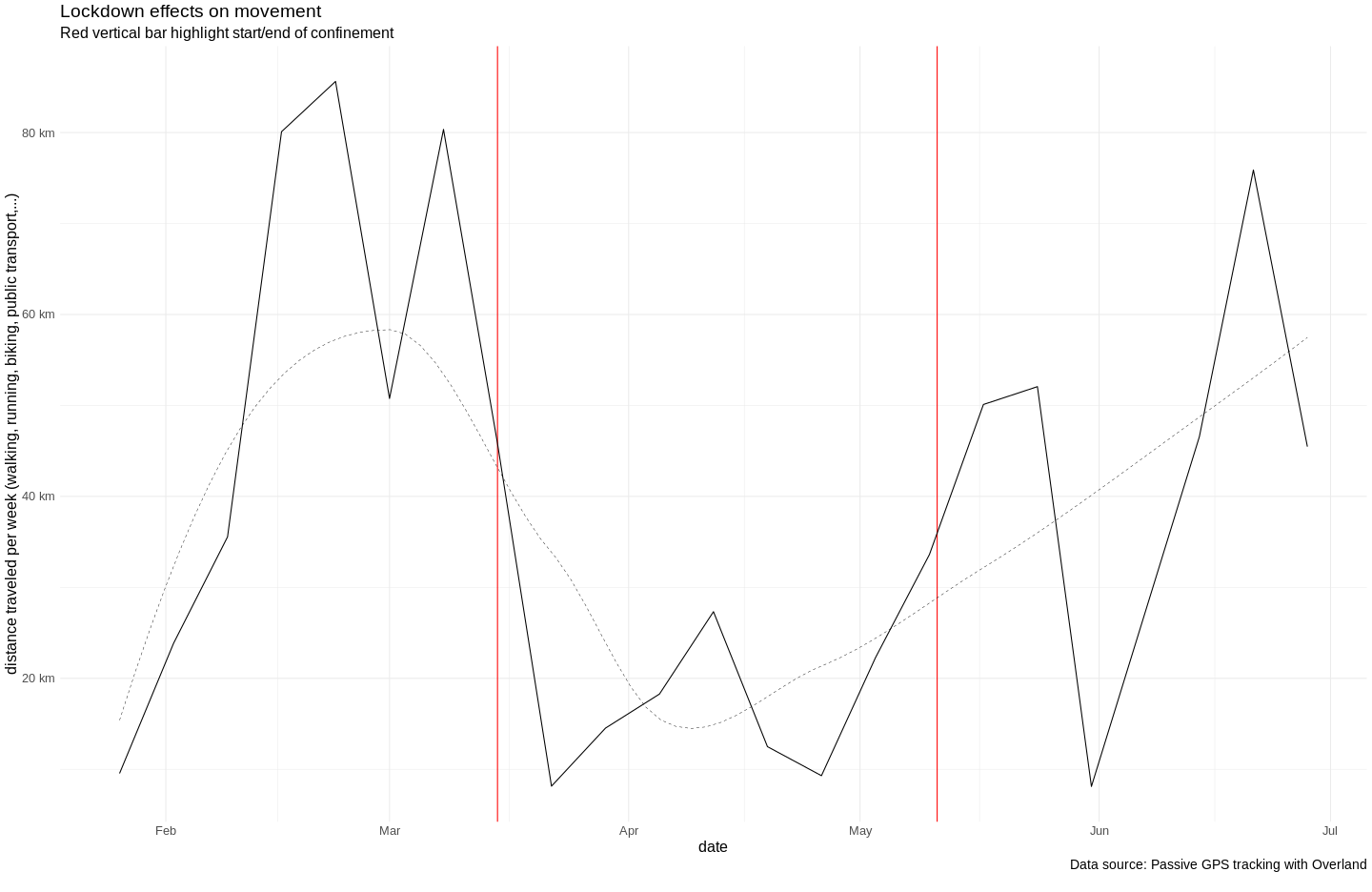# Tracking phone usage throughout the Lockdown¶

Moment doesn't have an API, so you need to have uploaded your `moment.json` file to the main folder of your notebook server.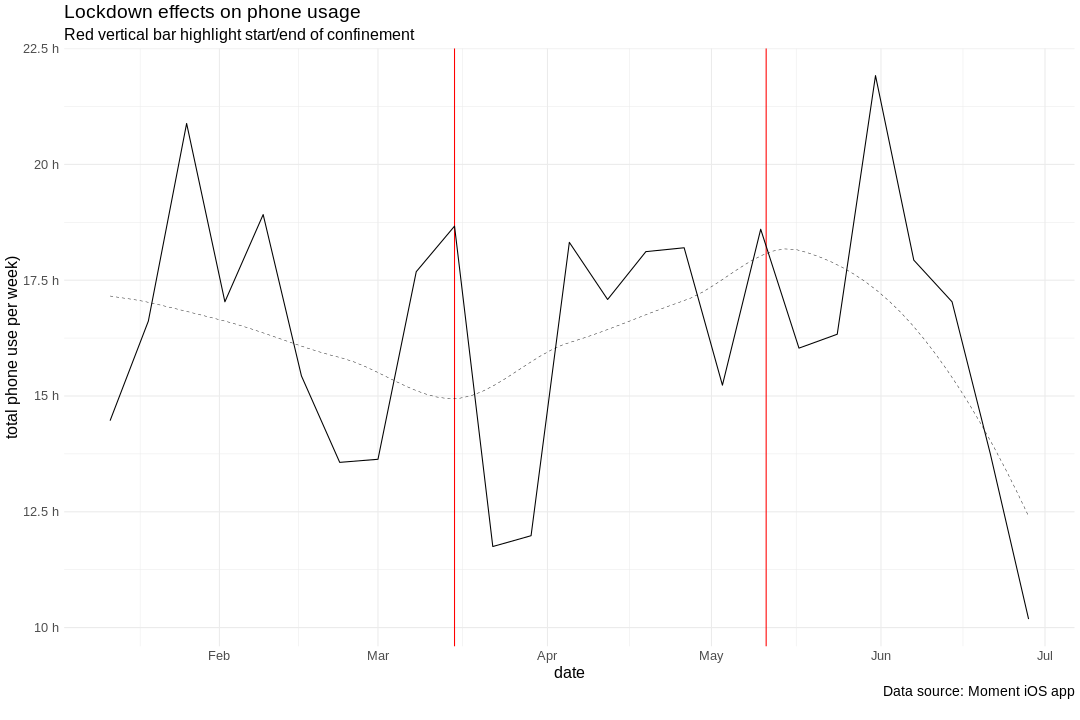# Lockdown effects¶

If you want to run this notebook and run into problems or have questions: Reach out to Bastian on Twitter or Slack

France was on COVID-19 lockdown from mid-March until mid-May. How did my behaviour change over those two months? Let's have a look at data on

• productivity
• physical activity
• sleep
• music listening habits
• movement behaviour

This notebook makes use of data from a variety of sources. If you want to run this analysis for your data you need the following data sources connected to your Open Humans account (or selectively run it):

In :
```### GET DATA FOR RESCUETIME, OURA AND SPOTIFY

from ohapi import api
import os
import requests
import tempfile
import json
import pandas as pd
from datetime import datetime

oura_present = ""
rescuetime_present = ""
spotify_present = ""

df_moment = ""
dataframe_oura_full = ""
rt_df_full = ""
df_spotify = ""
overland_subset = ""

user_details = api.exchange_oauth2_member(os.environ.get('OH_ACCESS_TOKEN'))
for i in user_details['data']:
if i['source'] == 'direct-sharing-184' and i['basename'] == 'oura-data.json':
oura_present = "True"
if i['source'] == "direct-sharing-149":
rescuetime_present = "True"
if i['source'] == 'direct-sharing-176' and i['basename'] == 'spotify-listening-archive.json':
spotify_present = "True"
if i['source'] == 'direct-sharing-176' and i['basename'] == 'spotify-track-metadata.json':
```
In :
```### PARSERS FOR OURA, SPOTIFY & RESCUETIME

dates = []
values = []
value_type = []

for sdate in oura['sleep']:
dates.append(sdate['summary_date'])
values.append(sdate['score'])
value_type.append('sleep')
dates.append(sdate['summary_date'])
values.append(sdate['total'])
value_type.append('sleep_sum')

for sdate in oura['activity']:
dates.append(sdate['summary_date'])
values.append(sdate['score'])
value_type.append('activity')
dates.append(sdate['summary_date'])
values.append(sdate['steps'])
value_type.append('steps')

dates.append(sdate['summary_date'])
values.append(sdate['score'])

dataframe = pd.DataFrame(
data = {
'date': dates,
'value': values,
'type': value_type
}
)
return dataframe

date = []
time_spent_seconds = []
activity = []
category = []
productivity = []
for element in rescuetime_data['rows']:
date.append(element)
time_spent_seconds.append(element)
activity.append(element)
category.append(element)
productivity.append(element)
date = [datetime.strptime(dt,"%Y-%m-%dT%H:%M:%S") for dt in date]

rt_df = pd.DataFrame(data={
'date': date,
'time_spent_seconds': time_spent_seconds,
'activity': activity,
'category': category,
'productivity': productivity
})
return rt_df

def parse_timestamp(lst):
timestamps = []
for item in lst:
try:
timestamp = datetime.strptime(
item,
'%Y-%m-%dT%H:%M:%S.%fZ')
except ValueError:
timestamp = datetime.strptime(
item,
'%Y-%m-%dT%H:%M:%SZ')
timestamps.append(timestamp)
return timestamps

track_title = []
artist_name = []
album_name = []
played_at = []
popularity = []
duration_ms = []
explicit = []
track_id = []

danceability = []
energy = []
key = []
loudness = []
mode = []
speechiness = []
acousticness = []
instrumentalness = []
liveness = []
valence = []
tempo = []

['danceability', 'energy', 'key', 'loudness', 'mode', 'speechiness', 'acousticness', 'instrumentalness', 'liveness', 'valence','tempo']

key_translation = {
'0': "C",
"1": "C#",
"2": "D",
"3": "D#",
"4": "E",
"5": "F",
"6": "F#",
"7": "G",
"8": "G#",
"9": "A",
"10": "A#",
"11": "B"
}

mode_translation = {'1':'major', '0': 'minor'}

for sp in sp_data:
track_title.append(sp['track']['name'])
artist_name.append(sp['track']['artists']['name'])
album_name.append(sp['track']['album']['name'])
played_at.append(sp['played_at'])
popularity.append(sp['track']['popularity'])
duration_ms.append(sp['track']['duration_ms'])
explicit.append(sp['track']['explicit'])
track_id.append(sp['track']['id'])

played_at = parse_timestamp(played_at)

dataframe = pd.DataFrame(data={
'track_id': track_id,
'track': track_title,
'artist': artist_name,
'album': album_name,
'popularity': popularity,
'duration_ms': duration_ms,
'explicit': explicit,
'played_at': played_at,
'danceability': danceability,
'energy': energy,
'key': key,
'loudness': loudness,
'mode': mode,
'speechiness': speechiness,
'acousticness': acousticness,
'instrumentalness': instrumentalness,
'liveness': liveness,
'valence': valence,
'tempo': tempo

})
dataframe = dataframe
return dataframe
```
In :
```### CREATE DATAFRAMES
if oura_present:
if rescuetime_present:
if spotify_present:
```

## Plotting the effects¶

There will be some inherent noise in day-to-day measures as behaviour changes depending on the day of the week (just think of weekdays vs weekends) To remove a good bit of this variance I'm taking only the weekly mean values (in case of sleep/activity) or the sum of all values within a week (in case of productivity).

In :
```%load_ext rpy2.ipython
```
```/opt/conda/lib/python3.7/site-packages/rpy2/robjects/pandas2ri.py:14: FutureWarning: pandas.core.index is deprecated and will be removed in a future version.  The public classes are available in the top-level namespace.
from pandas.core.index import Index as PandasIndex
```
In :
```%%R -i dataframe_oura_full,rt_df_full,df_spotify,oura_present,spotify_present,rescuetime_present -w 10 -h 10 --units in

## here we load the R packages and submit our processed data to the R kernel which will take care of all the rest
library(lubridate)
library(ggplot2)
if (!'cowplot' %in% installed.packages()) install.packages('cowplot',repos = "http://cran.us.r-project.org")
library(cowplot)
if (oura_present != ""){
dataframe_oura_full\$date <- as.Date(dataframe_oura_full\$date)
dataframe_oura_full\$week <- floor_date(dataframe_oura_full\$date,unit='week')
df_oura_agg_full <- aggregate(value~week+type,data=dataframe_oura_full,FUN=mean)
}

if (rescuetime_present != ""){
rt_df_full\$hour <-hour(rt_df_full\$date)
rt_df_full <- subset(rt_df_full, rt_df_full\$productivity >= 1)
rt_df_full\$date <- as.Date(rt_df_full\$date)
rt_df_full\$week <- floor_date(rt_df_full\$date,unit='week')
}

if (spotify_present != ""){
df_spotify\$date <- as.Date(df_spotify\$played_at)
df_spotify\$week <- floor_date(df_spotify\$date,unit='week')
df_spotify_agg <- aggregate(duration_ms~week,data=df_spotify,FUN=sum)
}
```
```WARNING:rpy2.rinterface_lib.callbacks:R[write to console]:
Attaching package: ‘lubridate’

WARNING:rpy2.rinterface_lib.callbacks:R[write to console]: The following objects are masked from ‘package:base’:

date, intersect, setdiff, union

WARNING:rpy2.rinterface_lib.callbacks:R[write to console]:
********************************************************

WARNING:rpy2.rinterface_lib.callbacks:R[write to console]: Note: As of version 1.0.0, cowplot does not change the

WARNING:rpy2.rinterface_lib.callbacks:R[write to console]:   default ggplot2 theme anymore. To recover the previous

WARNING:rpy2.rinterface_lib.callbacks:R[write to console]:   behavior, execute:
theme_set(theme_cowplot())

WARNING:rpy2.rinterface_lib.callbacks:R[write to console]: ********************************************************

WARNING:rpy2.rinterface_lib.callbacks:R[write to console]:
Attaching package: ‘cowplot’

WARNING:rpy2.rinterface_lib.callbacks:R[write to console]: The following object is masked from ‘package:lubridate’:

stamp

```
In :
```from IPython.display import Javascript, display
from ipywidgets import widgets
import datetime

def run_all(ev):
display(Javascript('IPython.notebook.execute_cells_below()'))

button = widgets.Button(description="Update plots!")

ld_start = widgets.DatePicker(
description='Start',
disabled=False,
value=datetime.datetime(2020,3,15).date()
)

ld_end = widgets.DatePicker(
description='End',
disabled=False,
value=datetime.datetime(2020,5,11).date()
)

boundaries = widgets.IntSlider(
value=10,
min=2,
max=16,
step=1,
description='Weeks before/after lockdown:',
disabled=False,
orientation='horizontal',
)

button.on_click(run_all)
display(ld_start,ld_end,boundaries)
```
In :
```display(button)
```

Warning: These widgets currently don't work in the Voila display as they use a hacky Javascript solution to re-run the notebook. If you want to edit those values you unfortunately have to do so from the regular Jupyter Notebook interface

In :
```START_DATE = str(ld_start.value)
END_DATE = str(ld_end.value)
WEEKS_BOUNDING = boundaries.value
```

## Effect on Productivity (as measured by RescueTime)¶

We start off by plotting the RescueTime data. RescueTime gives us some details on which categories the time is spent in, but we'll define some extra categories, which are meetings (via Zoom, Google Meet, Jitsi etc. as well as in-person meetings) and messages, which would be Slack, Mail, Rocket.Chat etc.

In :
```%%R -i START_DATE,END_DATE,WEEKS_BOUNDING -w 15 -h 10 --units in
if(rescuetime_present != ''){
rt_df <- subset(rt_df_full, rt_df_full\$week < as.Date(END_DATE) + weeks(WEEKS_BOUNDING))
rt_df <- subset(rt_df, rt_df\$week > as.Date(START_DATE) - weeks(WEEKS_BOUNDING))
rt_df_agg <- aggregate(time_spent_seconds~week+activity,data=rt_df,FUN=sum)

rt_df_agg_all <- aggregate(time_spent_seconds~week,data=rt_df,FUN=sum)

meeting_subset_df <- subset(rt_df, rt_df\$activity %in% meeting_activities)
rt_df_agg_meetings <- aggregate(time_spent_seconds~week,data=meeting_subset_df, FUN=sum)

message_activities = c('Slack', 'Mail','rocket.chat')
message_subset_df <- subset(rt_df, rt_df\$activity %in% message_activities)
rt_df_agg_message <- aggregate(time_spent_seconds~week,data=message_subset_df, FUN=sum)

ggplot(rt_df_agg_all,aes(x=week,y=time_spent_seconds/60/60)) +
geom_vline(xintercept=as.Date(END_DATE), color='red') +
geom_vline(xintercept=as.Date(START_DATE), color='red') +
geom_line() +
geom_smooth(se=FALSE,color='black',linetype = "dashed",size=0.2,method='loess',formula='y ~ x') +
geom_line(data=rt_df_agg_meetings,color='#b2df8a') +
geom_smooth(data=rt_df_agg_meetings,se=FALSE,color='#b2df8a',linetype = "dashed",size=0.2,method='loess',formula='y ~ x') +
geom_line(data=rt_df_agg_message,color='#1f78b4') +
geom_smooth(data=rt_df_agg_message,se=FALSE,color='#1f78b4',linetype = "dashed",size=0.2,method='loess',formula='y ~ x') +
theme_minimal() +
scale_x_date("date") +
scale_y_continuous("per week",labels = function(x) paste0(x, "h")) +
labs(
title = "Lockdown effects as measured by RescueTime",
subtitle = "Red vertical bar highlight start/end of confinement",
caption = 'Black line: Total weekly work hours.\nGreen line: Meetings (Google Meet, Zoom, in person...)\nBlue line: Messaging (Slack, eMail, ...)\n'
) + theme(text = element_text(size=16)) +
theme(plot.caption= element_text(size=14))
}
```The plot divides the time in before and after the lockdown, with everything in between the two red bars being the lockdown period. The dashed lines are smoothed, while the continuous lines give the individual weekly data points. There are some clear differences to be seen.

# Effect on Activity & Sleep (Oura)¶

Let's also check out the sleep and physical activity for the lockdown, here taken from the Oura ring.

In :
```%%R  -w 15 -h 8 --units in
if (oura_present != ""){
step_plot <- ggplot(subset(df_oura_agg_full, df_oura_agg_full\$week > as.Date(START_DATE) - weeks(WEEKS_BOUNDING) & df_oura_agg_full\$week < as.Date(END_DATE) + weeks(WEEKS_BOUNDING) & as.character(df_oura_agg_full\$type) %in% c('steps')), aes(x=week,y=value/1000)) +
geom_vline(xintercept=as.Date(START_DATE), color='red') +
geom_vline(xintercept=as.Date(END_DATE), color='red') +
geom_line() + theme_minimal() +
geom_smooth(se = FALSE,color='grey',method='loess',formula='y ~ x') +
scale_y_continuous(" ",labels = function(x) paste0(x, "k")) +
facet_grid(type ~ .) + labs(
) + theme(text = element_text(size=15)) +
theme(plot.caption= element_text(size=9))

sleep_plot <- ggplot(subset(df_oura_agg_full, df_oura_agg_full\$week > as.Date(START_DATE) - weeks(WEEKS_BOUNDING) & df_oura_agg_full\$week < as.Date(END_DATE) + weeks(WEEKS_BOUNDING) & as.character(df_oura_agg_full\$type) %in% c('sleep_sum')), aes(x=week,y=value/60/60)) +
geom_vline(xintercept=as.Date(START_DATE), color='red') +
geom_vline(xintercept=as.Date(END_DATE), color='red') +
geom_line() + theme_minimal() +
geom_smooth(se = FALSE,color='grey',method='loess',formula='y ~ x') +
scale_y_continuous(" ",labels = function(x) paste0(x, "h")) +
facet_grid(type ~ .) + labs(
) + theme(text = element_text(size=15)) +
theme(plot.caption= element_text(size=9))

score_plot <- ggplot(subset(df_oura_agg_full, df_oura_agg_full\$week > as.Date(START_DATE) - weeks(WEEKS_BOUNDING) & df_oura_agg_full\$week < as.Date(END_DATE) + weeks(WEEKS_BOUNDING) & as.character(df_oura_agg_full\$type) %in% c('sleep','activity','readiness')), aes(x=week,y=value)) +
geom_vline(xintercept=as.Date(START_DATE), color='red') +
geom_vline(xintercept=as.Date(END_DATE), color='red') +
geom_line() + theme_minimal() +
geom_smooth(se = FALSE,color='grey',method='loess',formula='y ~ x') +
scale_y_continuous('score') +
facet_grid(type ~ .) + theme(text = element_text(size=15)) +
theme(plot.caption= element_text(size=9))

title <- ggdraw() +
draw_label(
"Lockdown effects as measured by Oura Ring.",
fontface = 'bold',
x = 0,
y= 0.8,
hjust = 0
) +
draw_label(
"Red bars highlight start/end of confinement in Paris",
x = 0,
y = 0.55,
hjust = 0
)+
draw_label(
"black lines: weekly averages, grey lines: loess fit",
x = 0,
y = 0.3,
hjust = 0
)

plot_grid(title,plot_grid(step_plot,sleep_plot,score_plot, ncol=3,  rel_heights = c(1,1,1)),nrow=2,rel_heights=c(0.1,1))
}
```We plot both the raw data for the average nightly sleep time and the average number of steps for a week and plot those, as well as some processed "scores" that Oura assigns, which range between 0-100, with 100 being "the best". Looks like the post-lockdown life is returning pretty much to normal. For good (in terms of physical activity) but also for bad (in terms of sleep)

# Did the confinement change how much I listen to music?¶

In :
```%%R  -w 20 -h 12 --units in
if (spotify_present != ""){
ggplot(subset(df_spotify_agg, df_spotify_agg\$week > as.Date(START_DATE) - weeks(WEEKS_BOUNDING) & df_spotify_agg\$week < as.Date(END_DATE) + weeks(WEEKS_BOUNDING)), aes(x=week,y=duration_ms/1000/60/60)) +
geom_vline(xintercept=as.Date(START_DATE), color='red') +
geom_vline(xintercept=as.Date(END_DATE), color='red') +
geom_line() + theme_minimal() +
geom_smooth(se = FALSE,color='grey',method='loess',formula='y ~ x') +
scale_y_continuous("hours of music per week") +
labs(
title = "Lockdown effects on music listening",
subtitle = "Red vertical bar highlight start/end of confinement",
caption = 'Data source: Spotify listening history (spotify.openhumans.org)\n'
) +
theme(text = element_text(size=16)) +
theme(plot.caption= element_text(size=14))
}
```# Effects on Movement¶

Thanks to Overland I also have a full history of how much I moved, regardless of the mode of transportation (walking, biking, public transport, …). How does the lockdown effect appear there?

In :
```### LOAD OVERLAND DATA OF THE RIGHT MONTHS, FILTER DOWN TO JUST COORDINATES & TIMESTAMP, MERGE INTO ONE BIG DF

import arrow
import geopy.distance
sd = [int(i) for i in START_DATE.split("-")]
ed = [int(i) for i in END_DATE.split("-")]

RANGE_START = arrow.get(sd,sd,sd).shift(weeks=-WEEKS_BOUNDING).format('YYYY-MM-DD')
RANGE_END = arrow.get(ed,ed,ed).shift(weeks=WEEKS_BOUNDING).format('YYYY-MM-DD')
overland_range = pd.date_range(RANGE_START,RANGE_END,
freq='MS').strftime("%Y-%m").tolist()

first_df = True
overland_present = ""

user_details = api.exchange_oauth2_member(os.environ.get('OH_ACCESS_TOKEN'))
for i in user_details['data']:
if i['basename'].startswith('overland-data-') and (i['basename'][14:21] in overland_range):
if first_df:
first_df = False
else:
if first_df == False:
overland_df = overland_df[['longitude','latitude','timestamp']]
overland_df = overland_df.sort_values(by=['timestamp'])
### GET THE _NEXT_ RECORDING TO BE ABLE TO CALCULATE THE DISTANCE
overland_df['longitude_next'] = overland_df['longitude'].shift(-1)
overland_df['latitude_next'] = overland_df['latitude'].shift(-1)
### OK, LET'S NOT USE ALL DATA, IT's TOO MUCH FOR THE NOTEBOOKS. SAMPLE DOWN TO 20%
distance = []
overland_subset = overland_df.dropna().sample(frac=0.20)
for row in overland_subset.itertuples():
distance.append(geopy.distance.distance((row.latitude,row.longitude), (row.latitude_next,row.longitude_next)).km)
overland_subset['distance'] = distance
overland_present = "Yes"
```
In :
```%%R -i overland_subset,START_DATE,END_DATE,WEEKS_BOUNDING,overland_present -w 20 -h 13 --units in
if (overland_present != ""){
overland_subset\$date <- as.Date(overland_subset\$timestamp)
overland_subset\$week <- floor_date(overland_subset\$date,unit='week')

overland_subset2 <- subset(overland_subset, overland_subset\$week < as.Date(END_DATE) + weeks(WEEKS_BOUNDING))
overland_subset2 <- subset(overland_subset2, rt_df\$week > as.Date(START_DATE) - weeks(WEEKS_BOUNDING))
overland_agg <- aggregate(distance~week,data=overland_subset2,FUN=sum)

ggplot(overland_agg,aes(x=week,y=distance)) +
geom_vline(xintercept=as.Date(END_DATE), color='red') +
geom_vline(xintercept=as.Date(START_DATE), color='red') +
geom_line() +
geom_smooth(se=FALSE,color='black',linetype = "dashed",size=0.2,method='loess',formula='y ~ x') +
theme_minimal() +
scale_x_date("date") +
scale_y_continuous("distance traveled per week (walking, running, biking, public transport,...)",labels = function(x) paste0(x, " km")) +
labs(
title = "Lockdown effects on movement",
subtitle = "Red vertical bar highlight start/end of confinement",
caption = 'Data source: Passive GPS tracking with Overland\n'
) + theme(text = element_text(size=16)) +
theme(plot.caption= element_text(size=14))
}
```